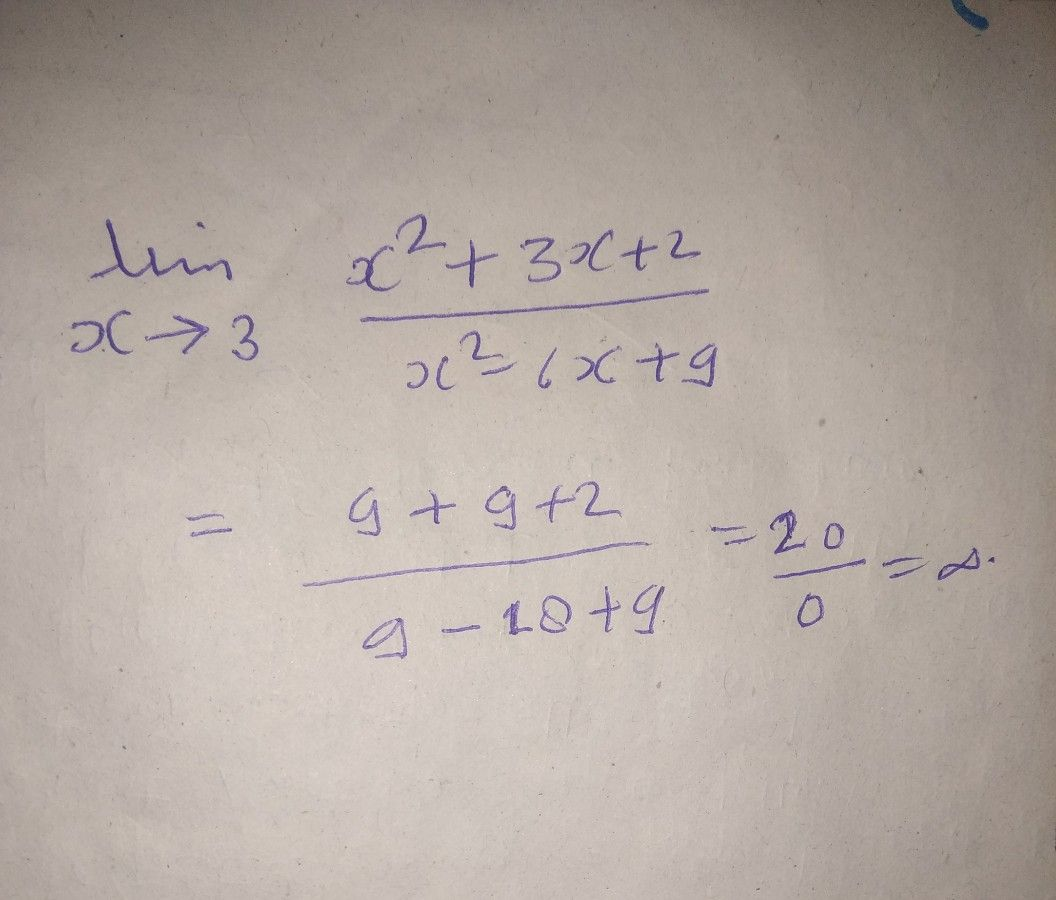Symbol
Problem$1$ $1.$ $lim _{x→3}\dfrac {x^{2}+3x+2} {x^{2}-6x+9}$
10th-13th grade
Calculus
SolutionQanda teacher - SushilStudent
ok
thanks sir